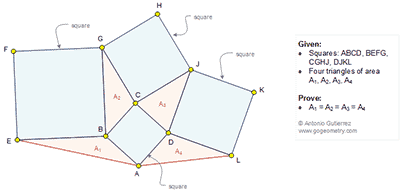## Sunday, March 6, 2016

### Geometry Problem 1197: Four Squares, Four Triangles, Equal Areas

Geometry Problem. Post your solution in the comment box below.
Level: Mathematics Education, High School, Honors Geometry, College.

Click the figure below to view more details of problem 1197.1.Note that ∡ (EBA) supplement to ∡ ( GBC) => sin (EBA)=sin(GBC)
A1= ½.EB.BA. sin (EBA)=1/2. BC.BG. sin(GBC)= A2
Similarly ∡ (BCG) supplement to ∡ ( DCJ) => sin (BCG)=sin(DCJ)
And A2=A3=1/2.BC.CG.sin(BCG)
And A3=A4=1/2.DC.DJ.sin(CDJ)
So A1=A2=A3=A4

2.Is angle GCB+ angle JCD=180 therefore A2=A3 (BC=CD, CG=CJ) and A3=A4 (CD=DA,DJ=DL, angle CDJ+angleADL=180)and A1=A2(BE=BG BA=BC angleEBA+angleGBC=1800.

3.Using the trigonometric formula for the area of any triangle XYZ, S(XYZ)= XY.YZ sin < XYZ and using another well known trigonometric identity sin@=sin(180-@)we have

A1 = BE.BA sin<ABE = BG.BC sin<CBG = A2 = BC.CG sin<BCG = CD.CJ sin <DCJ = A3 = CD.DJ sin CDJ = AD.DC sin ADC = A4

If we want to avoid geometry, we can drop perpendiculars from C to BG and A to BE extended and prove thro' congruence ASA that these perpendiculars are equal in length and hence A1 = A2.

Similarly by dropping perpendiculars G to BC and J to CD, A2 = A3 and by dropping perpendiculars from C to DJ and L to AD, A3 = A4

Hence by trigonometry or geometry A1 = A2 = A3 = A4

Sumith Peiris
Moratuwa
Sri Lanka

1.In the 3rd paragraph I should have said

"If we want to avoid trigonometry"

4.Draw altitudes, GG' on BC, JJ' on CD, LL' on AD, EE' on AB
Right tr GCG'=JCJ' (perpend sides) =>GG'=JJ'=>A2=A3
Tr JDJ'=LDL' => A3=A4
Draw E'E"=CG' =>EBE"=BCG=AEB =>A1=A2